## Package installation

``````install.packages("devtools")
devtools::install_github("Simon-Leonard/FlexDotPlot")

``````

``````library(FlexDotPlot)
``````

## Run the dot_plot function

### 1. Example dataset {.tabset}

#### Command

``````data(PBMC3K_example_data)
``````

``````head(PBMC3K_example_data)
#>   genes.plot           id   pct.exp pct.exp2 pct.exp100    avg.exp
#> 1       LDHB CD4+ T cells 0.9244136     0.92         92 13.7873904
#> 2       CD3D CD4+ T cells 0.8696785     0.87         87 10.9083613
#> 3       CD3E CD4+ T cells 0.7688966     0.77         77  7.5569240
#> 4       IL7R CD4+ T cells 0.6620330     0.66         66  6.7337967
#> 5        MAL CD4+ T cells 0.2719374     0.27         27  1.7643244
#> 6     S100A9 CD4+ T cells 0.1589922     0.16         16  0.7389524
#>   avg.exp.scale abs_avg_exp_scale  avg_logFC avg_logFC2     p_val_adj
#> 1     2.2133094         2.2133094  1.1488046       1.15 2.014606e-230
#> 2     1.3521395         1.3521395  1.0446491       1.04 1.319434e-183
#> 3     1.5524263         1.5524263  0.9718669       0.97 2.800347e-139
#> 4     1.8077169         1.8077169  1.0676981       1.07 4.243063e-125
#> 5     2.4562299         2.4562299  0.9090779       0.91  7.404012e-72
#> 6    -0.4489301         0.4489301 -3.2925866      -3.29  4.984636e-99
#> 1  2,00E-230   <1e-100
#> 2   1.3e-183   <1e-100
#> 3   2.8e-139   <1e-100
#> 4   4.2e-125   <1e-100
#> 5    7.4e-72    <1e-50
#> 6   5,00E-99    <1e-50
``````

#### Input overview (summary)

``````summary(PBMC3K_example_data)
#>   genes.plot             id               pct.exp          pct.exp2
#>  Length:312         Length:312         Min.   :0.0000   Min.   :0.0000
#>  Class :character   Class :character   1st Qu.:0.0328   1st Qu.:0.0300
#>  Mode  :character   Mode  :character   Median :0.1120   Median :0.1100
#>                                        Mean   :0.2910   Mean   :0.2909
#>                                        3rd Qu.:0.4773   3rd Qu.:0.4800
#>                                        Max.   :1.0000   Max.   :1.0000
#>
#>    pct.exp100        avg.exp         avg.exp.scale     abs_avg_exp_scale
#>  Min.   :  0.00   Min.   :  0.0000   Min.   :-0.8368   Min.   :0.0207
#>  1st Qu.:  3.00   1st Qu.:  0.1398   1st Qu.:-0.5065   1st Qu.:0.3559
#>  Median : 11.00   Median :  0.5717   Median :-0.3759   Median :0.4567
#>  Mean   : 29.09   Mean   : 10.0988   Mean   : 0.0000   Mean   :0.6930
#>  3rd Qu.: 48.00   3rd Qu.:  4.6576   3rd Qu.:-0.1742   3rd Qu.:0.6398
#>  Max.   :100.00   Max.   :442.2696   Max.   : 2.4749   Max.   :2.4749
#>
#>  Min.   :-3.2926   Min.   :-3.2900   Min.   :0.000000   Length:312
#>  1st Qu.:-1.3714   1st Qu.:-1.3125   1st Qu.:0.000000   Class :character
#>  Median :-0.8549   Median : 0.7650   Median :0.000006   Mode  :character
#>  Mean   :-0.4376   Mean   : 0.5294   Mean   :0.391716
#>  3rd Qu.:-0.1265   3rd Qu.: 1.9550   3rd Qu.:1.000000
#>  Max.   : 5.9443   Max.   : 5.9400   Max.   :1.000000
#>                    NA's   :198
#>   pval_symb
#>  Length:312
#>  Class :character
#>  Mode  :character
#>
#>
#>
#>
``````

### 2. Choose variables to plot {.tabset}

#### Use the size parameters

Size can be set with a column name using the size_var argument. Size scale can be adjusted with the shape.scale argument

``````dot_plot(data.to.plot = PBMC3K_example_data, size_var = "pct.exp", shape.scale = 8)
#> Using :
#>  - pct.exp values to set shape size
#>  - Nothing to set shape color
#>  - Nothing to add text on shapes
``````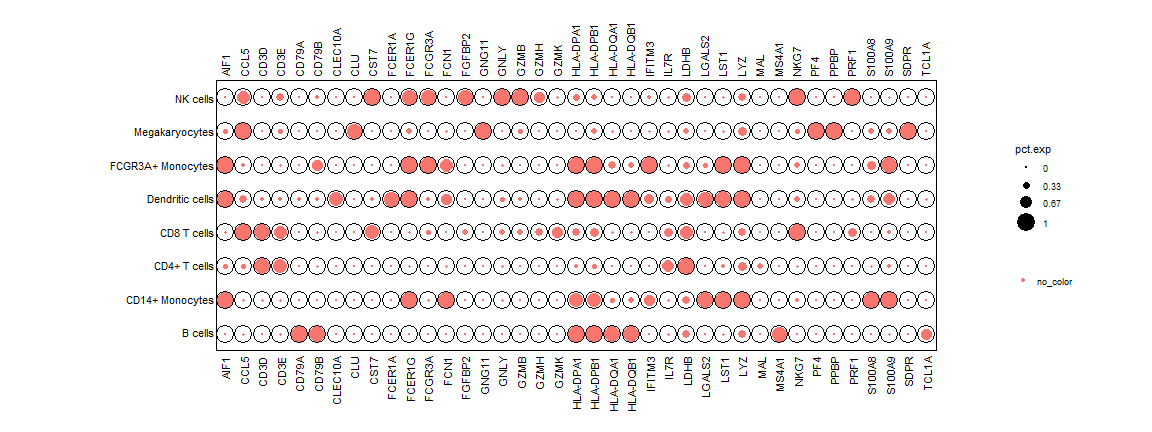A column index or a vector can also be provided

``````# Same results
dot_plot(data.to.plot = PBMC3K_example_data, size_var = 3, shape.scale = 8) # Third column = pct.exp
dot_plot(data.to.plot = PBMC3K_example_data, size_var = PBMC3K_example_data\$pct.exp, shape.scale = 8)
``````

Comment : The variable controlling the shape size has to be numeric

``````class(PBMC3K_example_data\$pval_symb)
dot_plot(data.to.plot = PBMC3K_example_data, size_var ="pval_symb") # Error ; size_var column has to be numeric
``````

Scale the size by size or radius with the scale.by argument (default = “radius”) Set lower and upper limits for scaling with scale.min and scale.max arguments, use NA for default values.

#### Use the color parameters

Color can be set with a column name using the col_var argument.

``````dot_plot(data.to.plot = PBMC3K_example_data, col_var = "pct.exp", shape.scale = 20)
#> Using :
#>  - Nothing to set shape size
#>  - pct.exp values to set shape color
#>  - Nothing to add text on shapes
``````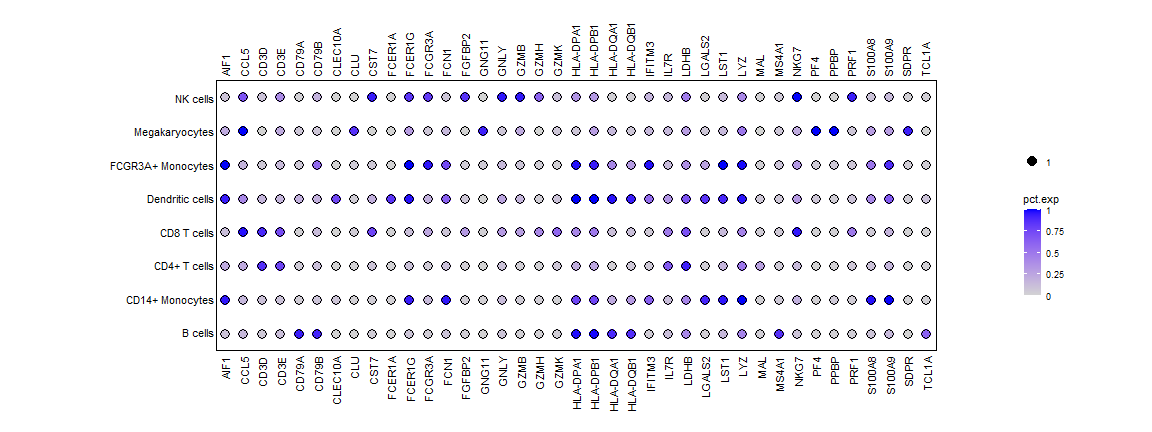A column index or a vector can also be provided

``````# Same results
dot_plot(data.to.plot = PBMC3K_example_data, col_var = 3, shape.scale = 20) # Third column = pct.exp
dot_plot(data.to.plot = PBMC3K_example_data, col_var = PBMC3K_example_data\$pct.exp, shape.scale = 20)
``````

Comment : A discrete variable can also be used to set the shape color

``````class(PBMC3K_example_data\$pval_symb)
#>  "character"
dot_plot(data.to.plot = PBMC3K_example_data, col_var ="pval_symb", shape.scale=20)
#> Using :
#>  - Nothing to set shape size
#>  - pval_symb values to set shape color
#>  - Nothing to add text on shapes
``````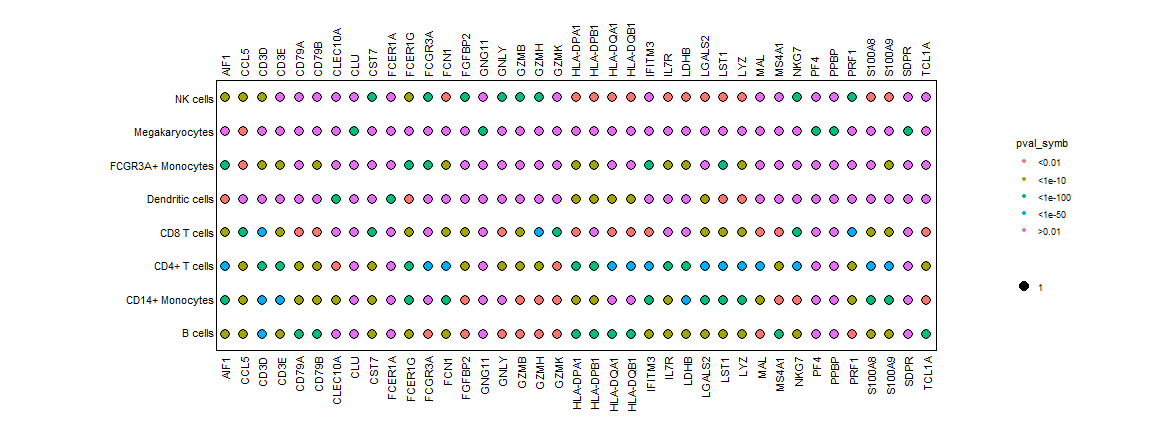##### Use custom colors

Custom colors can be used with the cols.use parameter

``````dot_plot(data.to.plot = PBMC3K_example_data, col_var = "pct.exp", cols.use = c("yellow","blue"), shape.scale = 20)
#> Using :
#>  - Nothing to set shape size
#>  - pct.exp values to set shape color
#>  - Nothing to add text on shapes
``````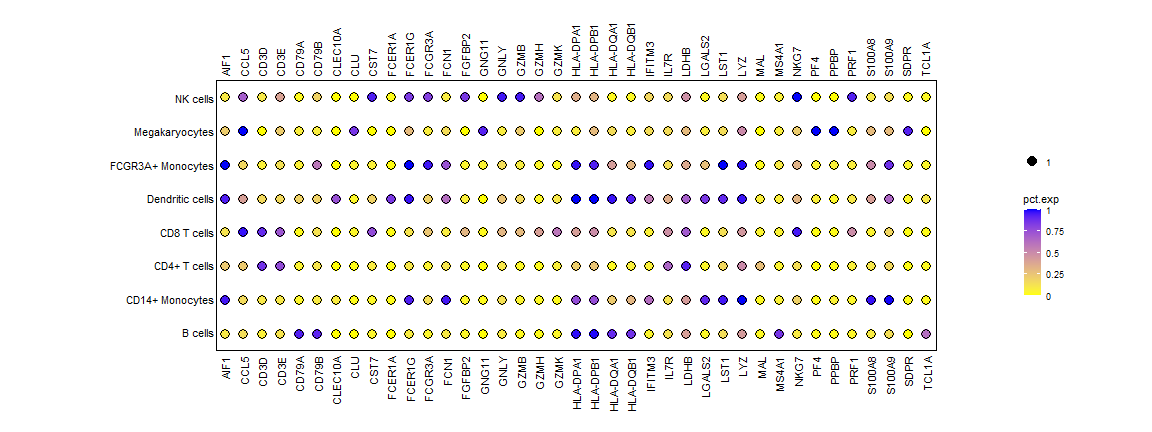#### Use the shape parameters

Variable shape can be set with a column name using the shape_var argument.

``````dot_plot(data.to.plot = PBMC3K_example_data, shape_var = "pct.exp", shape.scale = 20)
#> Using :
#>  - Nothing to set shape size
#>  - Nothing to set shape color
#>  - Nothing to add text on shapes
#>  - pct.exp values to determine shapes (204 shapes detected)
``````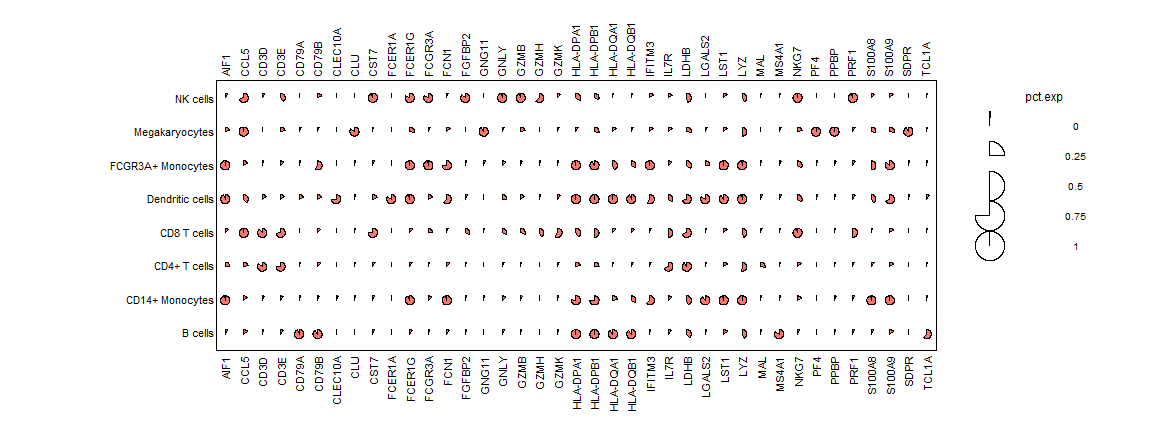A vector can also be provided

``````# Same results
dot_plot(data.to.plot = PBMC3K_example_data, shape_var = PBMC3K_example_data\$pct.exp, shape.scale = 8)
``````

Comment : A discrete variable can also be used to set the shape

``````class(PBMC3K_example_data\$pval_symb)
#>  "character"
dot_plot(data.to.plot = PBMC3K_example_data, shape_var ="pval_symb", shape.scale=20)
#> Using :
#>  - Nothing to set shape size
#>  - Nothing to set shape color
#>  - Nothing to add text on shapes
#>  - pval_symb values to determine shapes (5 shapes detected)
``````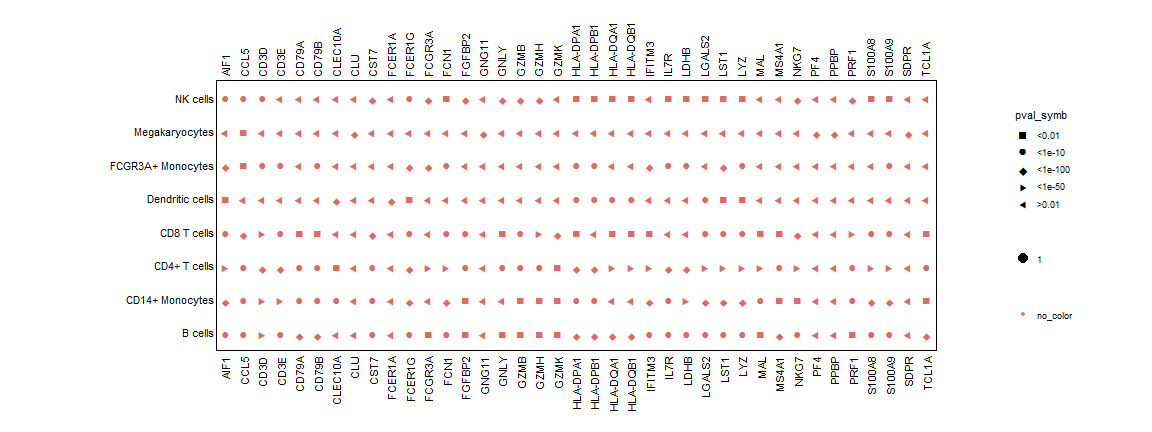You can choose the shape types with the shape_use argument and unicode characters

``````dot_plot(data.to.plot = PBMC3K_example_data, shape_var ="pval_symb", shape_use=c("\u2605","\u2736","\u25CF","\u2737","\u2726"),
shape.scale=20)
#> Using :
#>  - Nothing to set shape size
#>  - Nothing to set shape color
#>  - Nothing to add text on shapes
#>  - pval_symb values to determine shapes (5 shapes detected)
``````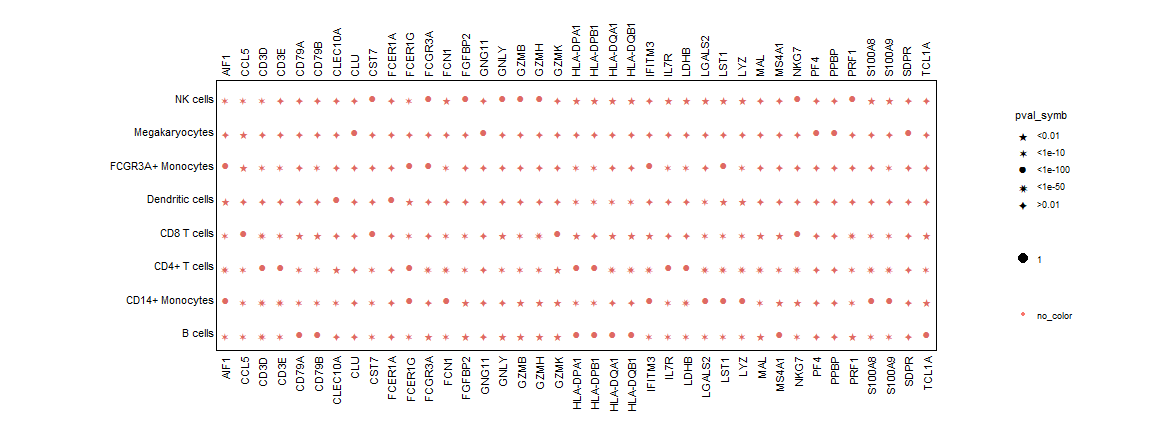Text can be set with a column name using the text_var argument. Text size can be adjusted with the text.size argument

``````dot_plot(data.to.plot = PBMC3K_example_data, text_var = "pct.exp2", text.size = 3, shape.scale = 20)
#> Using :
#>  - Nothing to set shape size
#>  - Nothing to set shape color
#>  - pct.exp2 values to add text on shapes
``````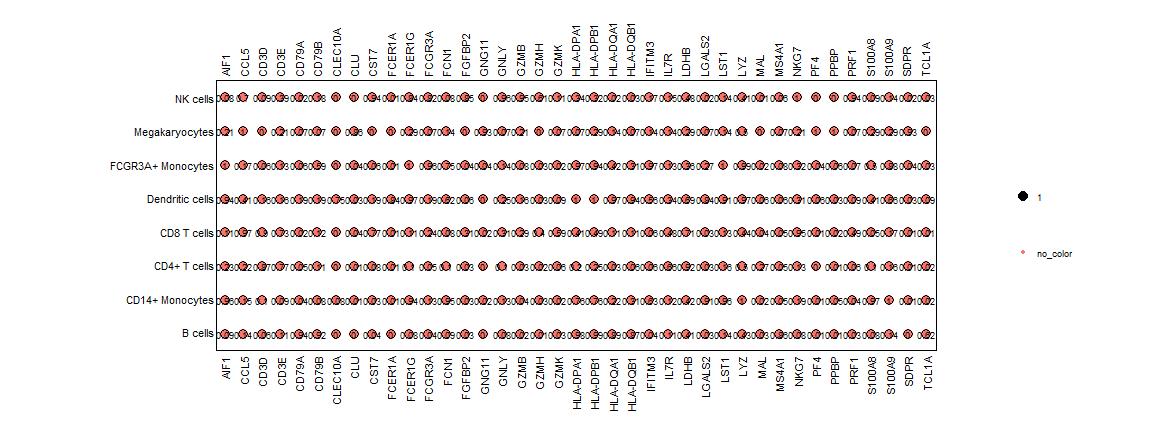A column index or a vector can also be provided

``````# Same results
dot_plot(data.to.plot = PBMC3K_example_data, text_var = 4, , text.size = 3, shape.scale = 20)
dot_plot(data.to.plot = PBMC3K_example_data, text_var = PBMC3K_example_data\$pct.exp2, , text.size = 3, shape.scale = 20)
``````

### 3. Combine variables

Shape, size, colors and text can be provided simultaneously and independently

``````dot_plot(data.to.plot = PBMC3K_example_data,
size_var = "pct.exp", shape.scale = 25,
shape_var= "pct.exp",
col_var = "avg_logFC"
)
#> Using :
#>  - pct.exp values to set shape size
#>  - avg_logFC values to set shape color
#>  - Nothing to add text on shapes
#>  - pct.exp values to determine shapes (204 shapes detected)
``````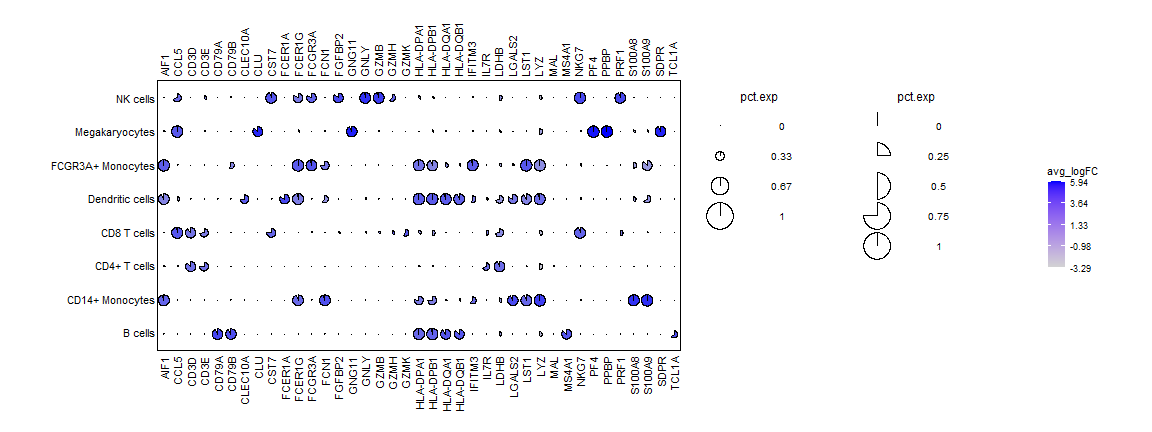``````
dot_plot(data.to.plot = PBMC3K_example_data,
size_var = "pct.exp", shape.scale = 8,
shape_var= ifelse(PBMC3K_example_data\$avg_logFC>0, "Up","Down"),
col_var = "avg_logFC", cols.use = c("yellow","blue")
)
#> Using :
#>  - pct.exp values to set shape size
#>  - avg_logFC values to set shape color
#>  - Nothing to add text on shapes
``````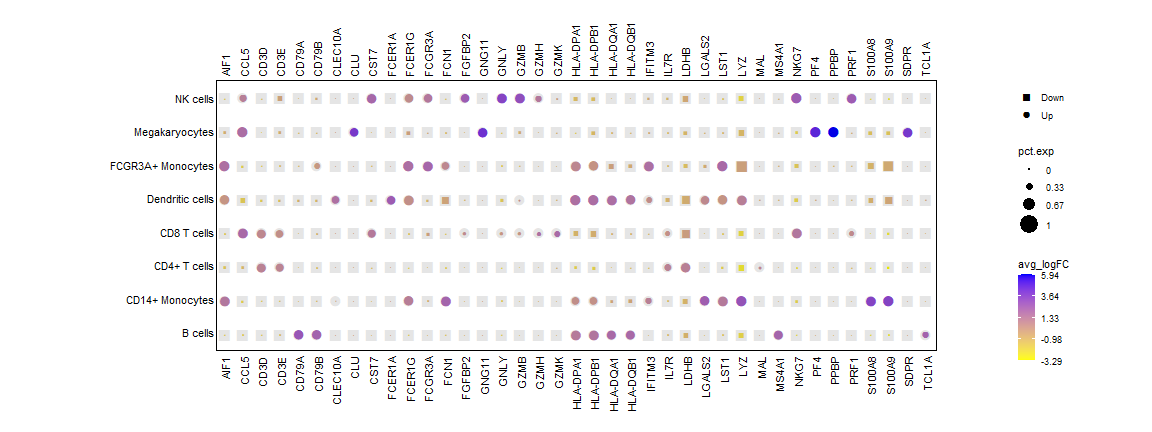### 4. Add dendrograms to the plot

Variable used to compute x and y dendrogramms can be provided within the dend_x_var and dend_y_var respectively. dist_method and hclust_methods arguments control distance method (default = euclidean) and hclust method (default = ward.D).

x dendrogramm only :

``````dot_plot(data.to.plot = PBMC3K_example_data,
size_var = "pct.exp", shape.scale = 25,
shape_var= "pct.exp",
col_var = "avg_logFC",
dend_x_var = c("pct.exp","avg_logFC"),
dist_method="euclidean", hclust_method="ward.D"
)
#> Using :
#>  - pct.exp values to set shape size
#>  - avg_logFC values to set shape color
#>  - Nothing to add text on shapes
#>  - pct.exp values to determine shapes (204 shapes detected) "In FAMD_x_var : Adding y index"
#>  "In FAMD_x_var : Adding x index"
``````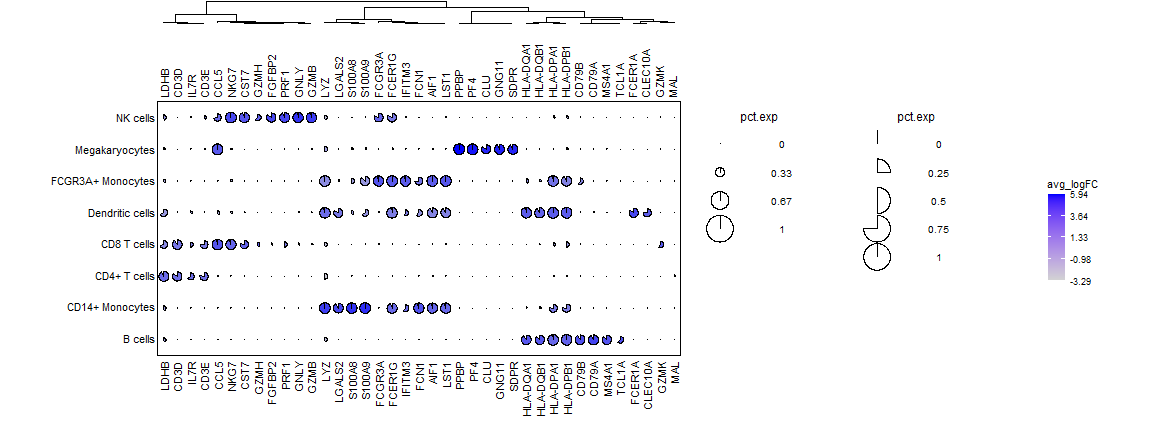x and y dendrogramms :

``````dot_plot(data.to.plot = PBMC3K_example_data,
size_var = "pct.exp", shape.scale = 25,
shape_var= "pct.exp",
col_var = "avg_logFC",
dend_x_var = c("pct.exp","avg_logFC"),
dend_y_var = c("pct.exp","avg_logFC"),
dist_method="euclidean", hclust_method="ward.D"
)
#> Using :
#>  - pct.exp values to set shape size
#>  - avg_logFC values to set shape color
#>  - Nothing to add text on shapes
#>  - pct.exp values to determine shapes (204 shapes detected) "In FAMD_x_var : Adding y index"
#>  "In FAMD_x_var : Adding x index"
#>  "In FAMD_y_var : Adding y index"
#>  "In FAMD_y_var : Adding x index"
``````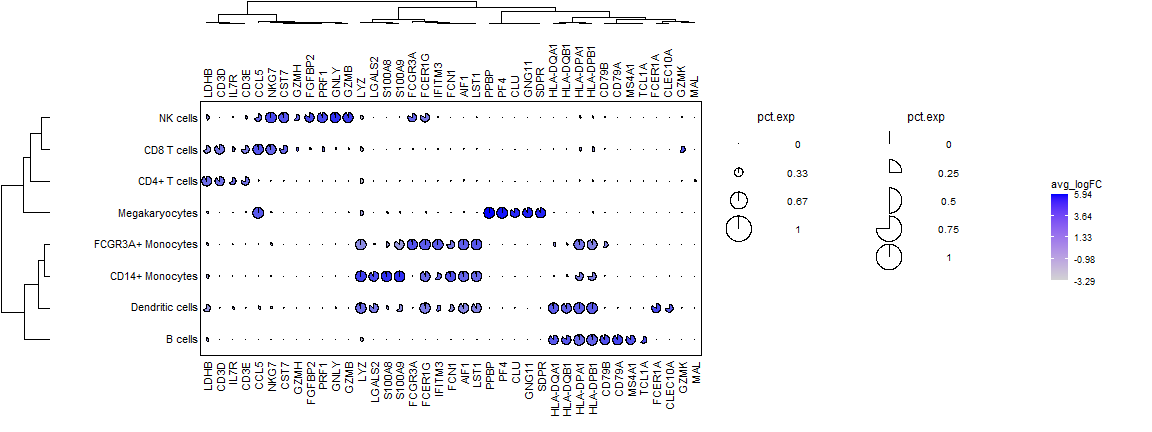### 5. Customize plot legends {.tabset}

#### Shape legend

Legend title can be modified with the size_legend, col_legend and shape_legend arguments.

``````dot_plot(data.to.plot = PBMC3K_example_data,
size_var = "pct.exp", shape.scale = 8, size_legend="My size legend",
shape_var= "pval_symb", shape_legend="My shape legend",
col_var = "avg_logFC", col_legend="My col legend"
)
#> Using :
#>  - pct.exp values to set shape size
#>  - avg_logFC values to set shape color
#>  - Nothing to add text on shapes
#>  - pval_symb values to determine shapes (5 shapes detected)
``````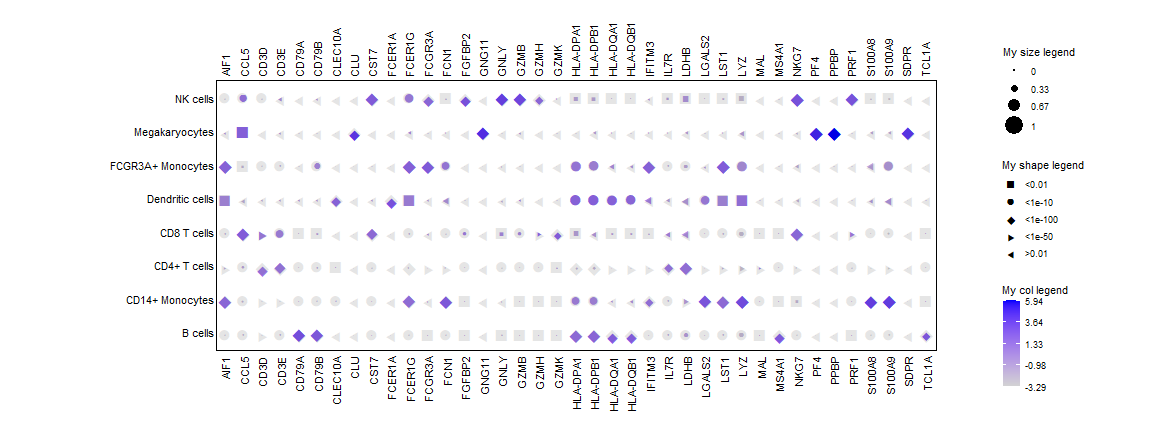If you don't want to plot the legend, set the plot.legend argument to FALSE.

``````dot_plot(data.to.plot = PBMC3K_example_data,
size_var = "pct.exp", shape.scale = 8,
shape_var= "pval_symb",
col_var = "avg_logFC",
plot.legend=F
)
#> Using :
#>  - pct.exp values to set shape size
#>  - avg_logFC values to set shape color
#>  - Nothing to add text on shapes
#>  - pval_symb values to determine shapes (5 shapes detected)
``````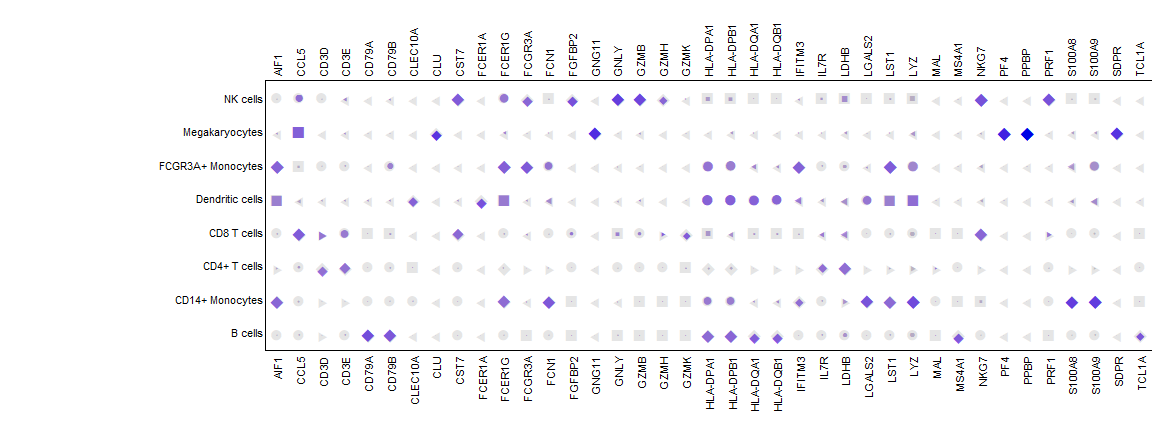size.breaks.number argument define the number of shapes with different size to display in the legend

``````dot_plot(data.to.plot = PBMC3K_example_data, size_var = "pct.exp", shape.scale = 8, size.breaks.number = 3)
#> Using :
#>  - pct.exp values to set shape size
#>  - Nothing to set shape color
#>  - Nothing to add text on shapes
``````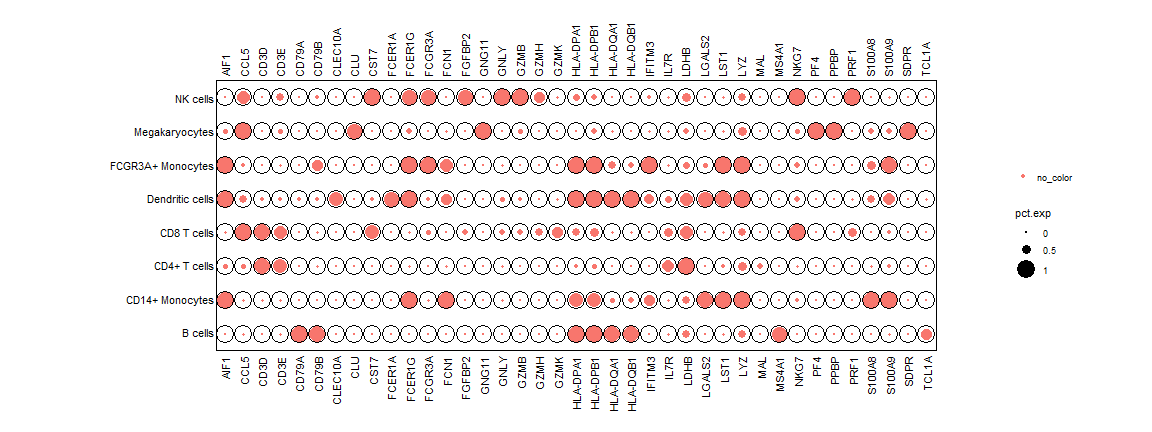You can also directly specify the values to get in the legend with the size.breaks.values argument

``````dot_plot(data.to.plot = PBMC3K_example_data, size_var = "pct.exp", shape.scale = 8, size.breaks.values = c(0,0.25,0.5,0.75,1))
#> Using :
#>  - pct.exp values to set shape size
#>  - Nothing to set shape color
#>  - Nothing to add text on shapesPutting size.breaks.values in size legend
``````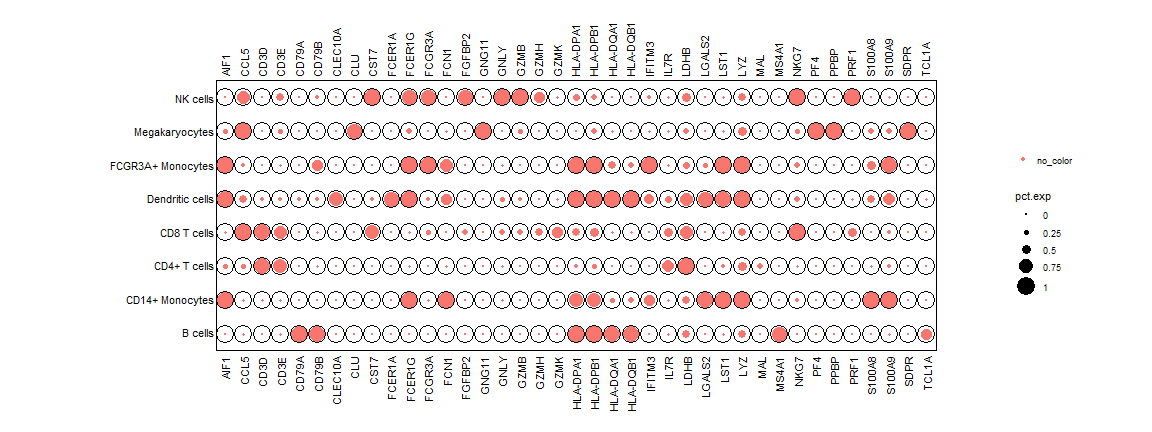color.breaks.number argument define the number of labels for the color gradient legend.

``````dot_plot(data.to.plot = PBMC3K_example_data, col_var = "pct.exp", shape.scale = 20, color.breaks.number = 3)
#> Using :
#>  - Nothing to set shape size
#>  - pct.exp values to set shape color
#>  - Nothing to add text on shapes
``````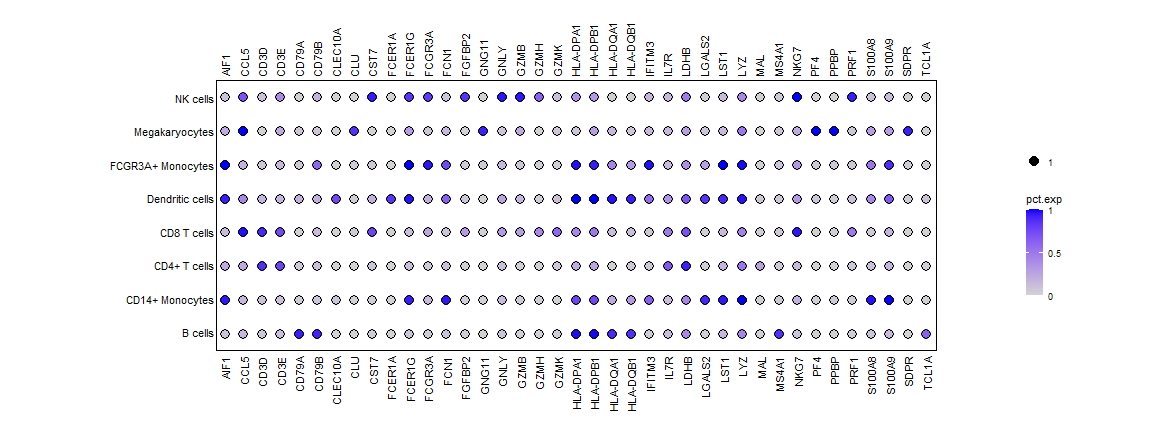You can also directly specify the values to get in the legend with the color.breaks.values argument

``````dot_plot(data.to.plot = PBMC3K_example_data, col_var = "pct.exp", shape.scale = 20, color.breaks.values = c(0,0.3,0.6,0.9))
#> Using :
#>  - Nothing to set shape size
#>  - pct.exp values to set shape color
#>  - Nothing to add text on shapes
#>  Putting color.breaks.values in color legend
``````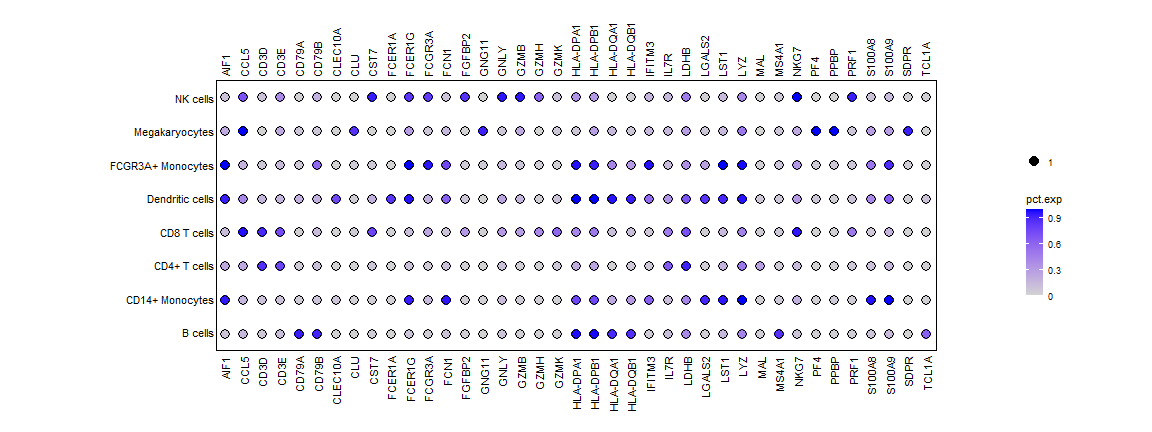#### Axis labels

Axis label positions can be controled with the x.lab.pos and y.lab.pos arguments. x.lab.pos must be one of “bottom”,“top”,“both” or “none”. y.lab.pos must be one of “left”,“right”,“both"or "none”.

``````dot_plot(data.to.plot = PBMC3K_example_data,
size_var = "pct.exp", shape.scale = 8,
shape_var= "pct.exp",
col_var = "avg_logFC",
x.lab.pos="bottom",
y.lab.pos="left"
)
#> Using :
#>  - pct.exp values to set shape size
#>  - avg_logFC values to set shape color
#>  - Nothing to add text on shapes
#>  - pct.exp values to determine shapes (204 shapes detected)
``````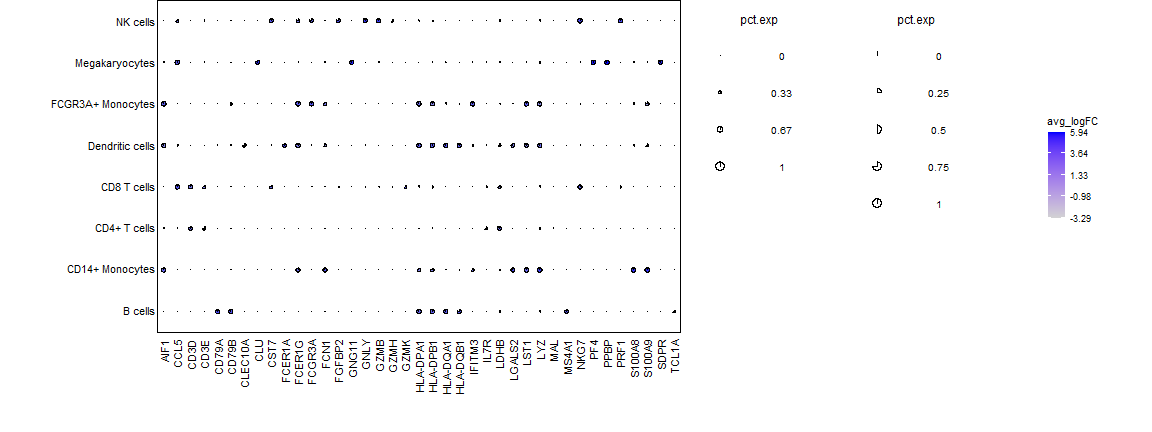Plot can be transposed by setting the transpose argument to TRUE

``````dot_plot(data.to.plot = PBMC3K_example_data,
size_var = "pct.exp", shape.scale = 8,
shape_var= "pct.exp",
col_var = "avg_logFC",
transpose=TRUE
)
#> Using :
#>  - pct.exp values to set shape size
#>  - avg_logFC values to set shape color
#>  - Nothing to add text on shapes
#>  - pct.exp values to determine shapes (204 shapes detected)
``````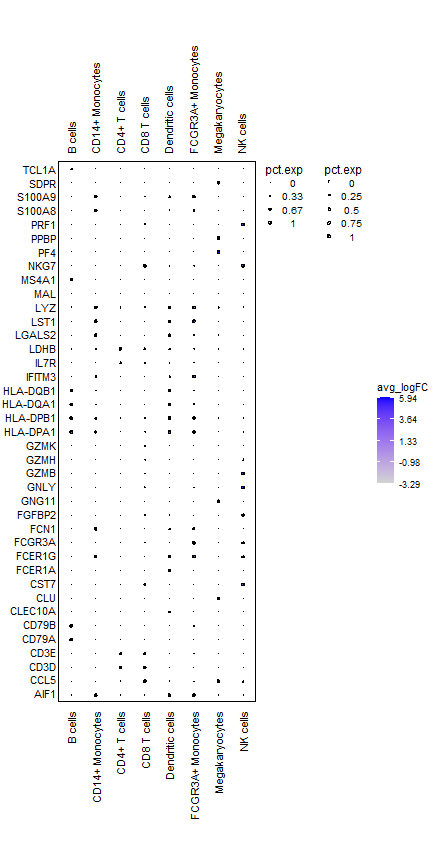### 6. Plot background {.tabset}

``````dot_plot(data.to.plot = PBMC3K_example_data,
size_var = "pct.exp", shape.scale = 25,
shape_var= "pct.exp",
col_var = "avg_logFC",
vertical_coloring=c(NA, "gray80")
)
#> Using :
#>  - pct.exp values to set shape size
#>  - avg_logFC values to set shape color
#>  - Nothing to add text on shapes
#>  - pct.exp values to determine shapes (204 shapes detected)
``````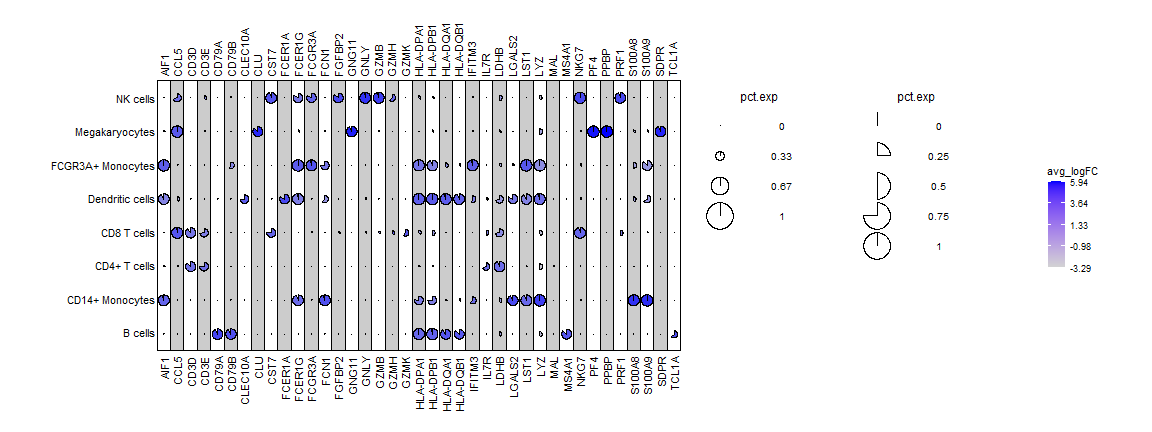``````dot_plot(data.to.plot = PBMC3K_example_data,
size_var = "pct.exp", shape.scale = 8,
shape_var= "pct.exp",
col_var = "avg_logFC",
horizontal_coloring=c(NA, "gray80")
)
#> Using :
#>  - pct.exp values to set shape size
#>  - avg_logFC values to set shape color
#>  - Nothing to add text on shapes
#>  - pct.exp values to determine shapes (204 shapes detected)
``````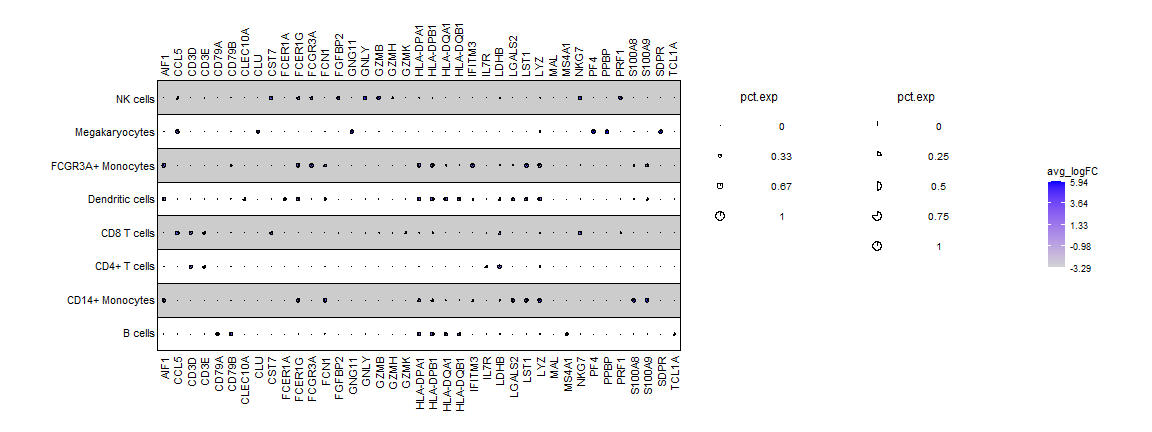### 7. Get the corresponding ggplot object

``````ggplot_object <- dot_plot(data.to.plot = PBMC3K_example_data,
size_var = "pct.exp", shape.scale = 8,
shape_var= "pct.exp",
col_var = "avg_logFC",
do.return = TRUE
)
``````

## Run the Shiny app

``````Shiny_dot_plot()
``````

## Reproduce the article figure

Full script to generate dotplot input can be found at in the FlexDotPlot_CBMC_dataset vignette

``````### Import data + create the exp.type column
data(CBMC8K_example_data)

### Dotplot
dotplot = dot_plot(
data.to.plot=CBMC8K_example_data,
shape_var="canonical_marker", shape_use = c("\u25CF","\u2737"),
x.lab.pos="bottom",y.lab.pos="left",
cols.use=c("lightgrey","orange","red", "darkred"),size_legend="RNA", col_legend="ADT", shape_legend="Canonical marker ?",
shape.scale =12, text.size=3,
plot.legend = TRUE,
size.breaks.number=4, color.breaks.number=4, shape.breaks.number=5
hclust_method="ward.D", do.return = TRUE)
#> Using :
#>  - RNA.avg.exp.scaled values to set shape size
#>  - ADT.avg.exp.scaled values to set shape color
#>  - canonical_marker values to determine shapes (2 shapes detected) "In FAMD_x_var : Adding y index"
#>  "In FAMD_x_var : Adding x index"
#>  "In FAMD_y_var : Adding y index"
#>  "In FAMD_y_var : Adding x index"
``````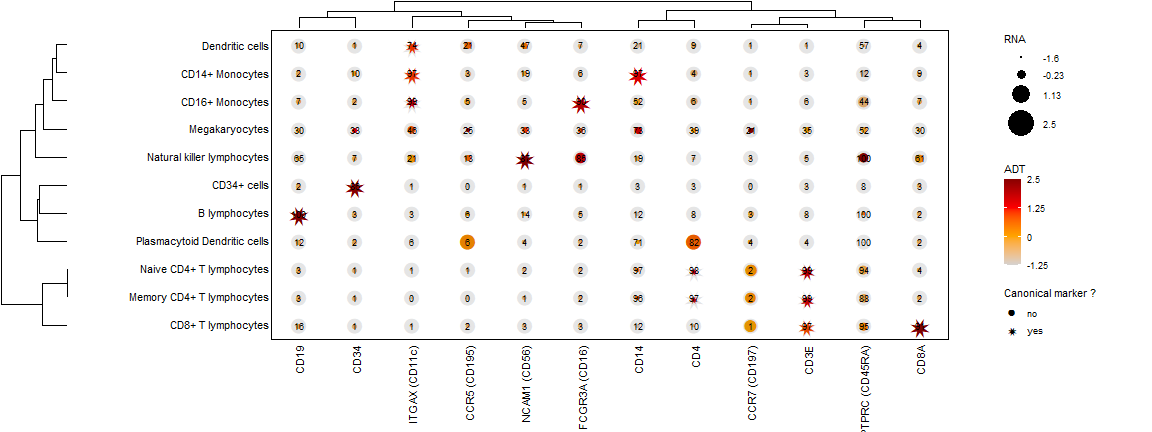## Session Info

``````#> R version 4.1.3 (2022-03-10)
#> Platform: x86_64-w64-mingw32/x64 (64-bit)
#> Running under: Windows 10 x64 (build 19044)
#>
#> Matrix products: default
#>
#> locale:
#>  LC_COLLATE=C                   LC_CTYPE=French_France.1252
#>  LC_MONETARY=French_France.1252 LC_NUMERIC=C
#>  LC_TIME=French_France.1252
#>
#> attached base packages:
#>  stats     graphics  grDevices utils     datasets  methods   base
#>
#> other attached packages:
#>  FlexDotPlot_0.2.2
#>
#> loaded via a namespace (and not attached):
#>   ggdendro_0.1.22      viridis_0.6.2        sass_0.4.0
#>   splines_4.1.3        jsonlite_1.7.2       viridisLite_0.4.0
#>   R.utils_2.11.0       bslib_0.3.1          shiny_1.7.1
#>  highr_0.9            ggrepel_0.9.1        pillar_1.6.4
#>  lattice_0.20-45      glue_1.5.1           digest_0.6.29
#>  promises_1.2.0.1     polyclip_1.10-0      colorspace_2.0-2
#>  R.oo_1.24.0          R.matlab_3.6.2       Matrix_1.4-0
#>  htmltools_0.5.2      httpuv_1.6.3         plyr_1.8.6
#>  FactoMineR_2.4       XML_3.99-0.8         pkgconfig_2.0.3
#>  grImport2_0.2-0      purrr_0.3.4          xtable_1.8-4
#>  scales_1.1.1         tweenr_1.0.2         jpeg_0.1-9
#>  later_1.3.0          ggforce_0.3.3        tibble_3.1.6
#>  mgcv_1.8-39          generics_0.1.1       farver_2.1.0
#>  ggplot2_3.3.5        ellipsis_0.3.2       DT_0.20
#>  cli_3.1.0            sisal_0.48           magrittr_2.0.1
#>  crayon_1.4.2         mime_0.12            evaluate_0.14
#>  R.methodsS3_1.8.1    fansi_0.5.0          nlme_3.1-155
#>  MASS_7.3-55          shinydashboard_0.7.2 colourpicker_1.1.1
#>  tools_4.1.3          lifecycle_1.0.1      stringr_1.4.0
#>  munsell_0.5.0        cluster_2.1.2        flashClust_1.01-2
#>  compiler_4.1.3       jquerylib_0.1.4      rlang_1.0.2
#>  grid_4.1.3           rstudioapi_0.13      htmlwidgets_1.5.4
#>  leaps_3.1            miniUI_0.1.1.1       labeling_0.4.2
#>  base64enc_0.1-3      shinyWidgets_0.6.2   boot_1.3-28
#>  gtable_0.3.0         reshape2_1.4.4       R6_2.5.1
#>  gridExtra_2.3        lubridate_1.8.0      knitr_1.36
#>  dplyr_1.0.8          fastmap_1.1.0        utf8_1.2.2
#>  bsplus_0.1.3         dendextend_1.15.2    stringi_1.7.6
#>  Rcpp_1.0.7           vctrs_0.3.8          png_0.1-7
#>  scatterplot3d_0.3-41 tidyselect_1.1.1     xfun_0.28
``````